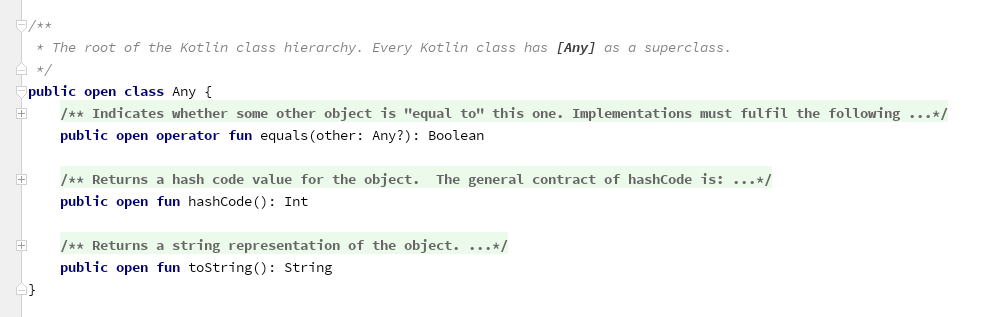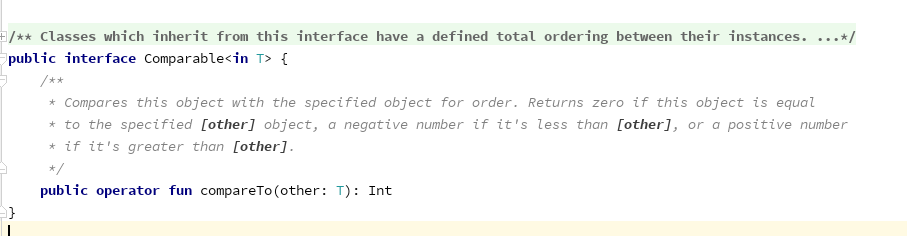### 重载算术运算符

#### 重载二元算术运算符

`a * b` `times`
`a / b` `div`
`a % b` `rem`,`mod(弃用)`
`a + b` `plus`
`a - b` `minus`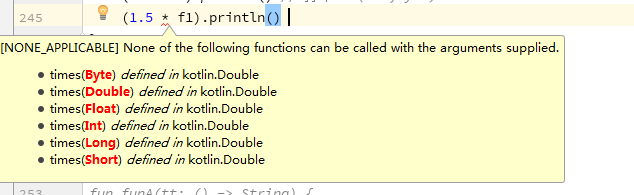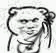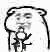#### 重载复合赋值运算符

`a += b` `timesAssign`
`a /= b` `divAssign`
`a %= b` `remAssign`
`a += b` `plusAssign`
`a -= b` `minusAssign`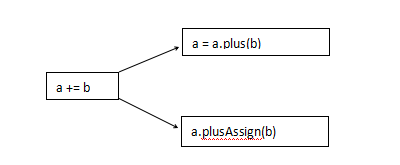• `+``-`运算符会返回一个新的集合。
• `+=``-=`用于可变集合，会修改集合，如果是只读，那么就会返回一个修改过的副本，也就是说只有在只读集合被定义为var类型的时候，才能使用`+=``-=`

#### 重载一元运算符

Kotlin中允许重载一元运算符，如`-a`,`+a`等等，同样我们列举支持的一元运算符和对应的函数名：

`+a` `unaryPlus`
`-a` `unaryMinus`
`!a` `not`
`++a, a++` `inc`
`--a, a--` `dec`

### 重载比较运算符

`a == b` `a?.equals(b) ?: (b === null)`
`a != b` `!(a?.equals(b) ?: (b === null))`
`a > b` `a.compareTo(b) > 0`
`a < b` `a.compareTo(b) < 0`
`a >= b` `a.compareTo(b) >= 0`
`a <= b` `a.compareTo(b) <= 0`

#### 等号运算符`equals`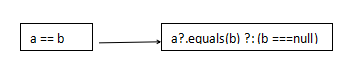#### 排序运算符`compareTo`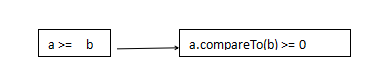`compareValuesBy`函数是按顺序依次调用回调方法，两两一组分别做比较，然后返回结果，如果则返回比较结果，如果相同，则继续调用下一个，如果没有更多回调来调用，则返回0。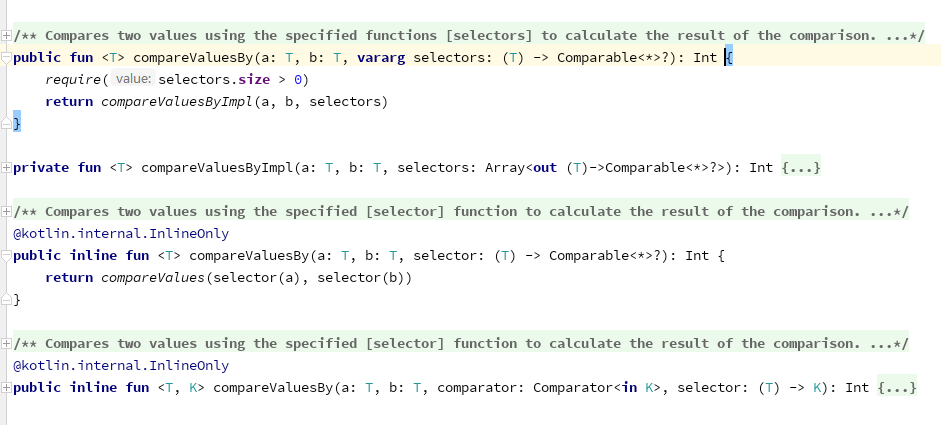#### `override`标记